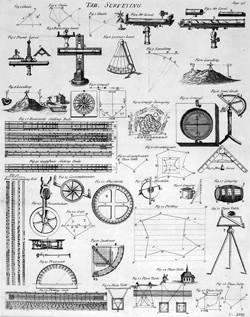Surveying is one of the oldest professions in the world, as ever since people started dividing up land into separate ownership there had to be someone to work out which bit belonged to whom. The earliest known surveyors were in Egypt, where annual floods from the river Nile meant that the boundary lines of fields had to be redrawn every year. Egyptian surveyors used marked lengths of rope and other simple tools combined with the rules of geometry you learn in school to measure distances, angles and areas, and although modern surveyors use high-tech infared beams instead of the Egyptians’ basic equipment, the mathematical principles remain unchanged.

### Totally surveyedA surveyor’s job is to determine the location of points in space, work out the relative distances between them, and produce a diagram or map. That might sound pretty simple, but think about trying to measure the length of the road you live on. If you’re on a hill then you have to be able to measure angles and use trigonometry to calculate an accurate position for the road’s two end points, or if the road is curved then you need to know how to calculate the length of a circular arc.

The main tool of the surveyor’s trade is the total station, a device combining a telescope and a system for measuring distances. Surveyors can work out the relative location of a target by looking at it through the telescope. The total station then displays the angle at which the telescope is rotated horizontally, using north as 0 degrees, and the angle at which it is rotated vertically, using the horizontal as 0 degrees. These two angles are enough to calculate the relative direction of the target, but determining its exact location requires a third number: the distance.

### There and back againThe total station’s electronic distance measuring device works by sending out an infrared beam to a reflective prism placed at the target by the surveyor, then timing how long it takes the beam to return. Infrared beams always travel at the speed of light, which is about 300,000,000 metres per second, often called c. If the beam takes t seconds to return then the distance travelled is c×t, but because the beam makes a return journey we have to half this to get the distance to the target, (c × t) / 2.

With the direction and distance of the target determined, the surveyor can use trigonometry to calculate the position of the target relative to the total station at the origin point. By repeating the procedure at a number of points, the surveyor can then calculate the lengths, areas and volumes that they need.

### Get out and do it

Surveyors play an important role in our society, helping us to construct buildings and roads, settle land ownership, and draw maps. In addition to strong mathematical skills, surveyors must also understand elements of engineering and the legal system. Life as a surveyor is quite varied, and much of the work takes place outdoors, so if you’re interested in becoming a surveyor, why not visit the website of the Royal Institution of Charted Surveyors for more information?